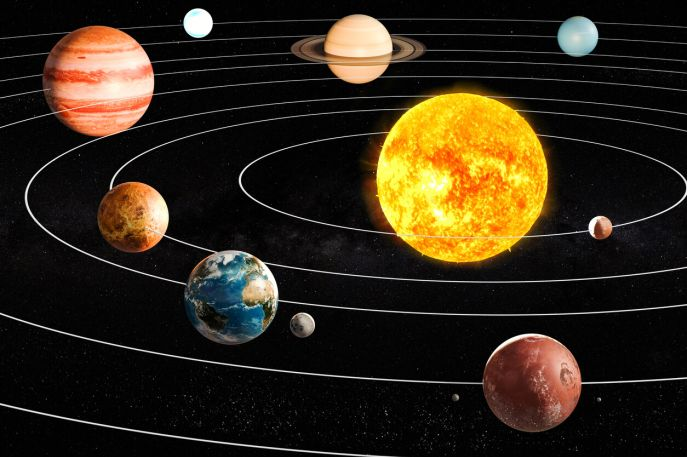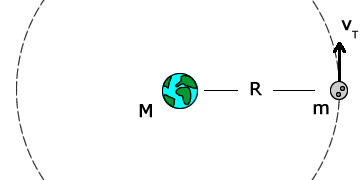# Orbital Motion Study Guide

Introduction

When we consider how incredibly huge the gorgeous sky above us can be, it’s hard to believe the enormity. As a fact, the Sun is the closest star to us out of about 200 billion trillion stars in the cosmos! When contrasted to other stars in the universe, it is a tiny star. The Sun and everything that orbits it make up our Solar System. Let’s take a closer look at orbital motion.Source

## ORBITAL MOTION

• We know that the meaning of motion is the change of the position of a body with time.

• This change in position is also observed in the orbital motion of the earth around the sun.

• The definition of orbital motion states that when a planetary body moves forward while being dragged by gravity toward another body, it is said to be in orbital motion.

• This motion can also be understood with the concept of how an object stays in a magnetic field.

• The meaning of magnetic moment is, in fact, the tendency of a body to align in a magnetic field.

• Gravitational interaction causes two bodies to orbit each other around their center of mass, with the less large mass following a bigger orbit.

• Outside these bodies or within the more large body, the center of mass can be found.

• The solar system’s center of mass is just outside the visible disc of the Sun.

## AN EXAMPLE OF THE ORBITAL MOTION OF THE MOON AROUND THE EARTH

• Since the moon is far closer to earth than the sun, it is influenced by earth’s gravity more than the sun’s.
• The moon is drawn closer to the earth by the gravitational attraction between the two bodies.
• At the same time, the moon has a forward motion that partially offsets the gravitational pull of the earth.
• As a result, the moon circles earth rather than dropping into the earth’s crust.Source

The orbital velocity is an important orbital motion equation that enables us to find the velocity at which a body revolves around another body. The orbital velocity is represented as

V orbit = √GM/ R

Where, G is the gravitational constant,

M is the mass of the body, and

R = radius of the orbit.

# SUMMARY

• Orbital motion is the motion of planetary bodies moving forward while being dragged by gravity toward another body.
• Gravitational interaction causes two bodies to orbit each other around their center of mass, with the less large mass following a bigger orbit.
• The orbital velocity is represented as V orbit = √GM/ R.

## FAQs

Q. What is orbital motion a combination of?

Orbital motion is a combination of a body’s forward velocity and the gravitational pull towards another body.

Q. What is the orbital motion of the galaxy?

The orbital motion of the galaxy is the rotational motion of the planetary bodies around another body, influenced by a forward velocity and a gravitational pull.

Q. What is circular orbital motion?

Circular orbital motion is the movement of the earth and other planetary bodies around the sun.

We hope you enjoyed studying this lesson and learned something cool about Orbital Motion! Join our Discord community to get any questions you may have answered and to engage with other students just like you! We promise, it makes studying much more fun!😎

## REFERENCE

1. Orbital Motion: https://www.ck12.org/physics/orbital-motion/lesson/Orbital-Motion-MS-PS/?referrer=concept_details. Accessed 11th April 2022.
2. Orbital Mechanics: https://en.wikipedia.org/wiki/Orbital_mechanics. Accessed 11th April 2022.
]]>